Courses

# NCERT Solutions(Part- 2)- Data Handling Class 8 Notes | EduRev

## Mathematics (Maths) Class 8

Created by: Full Circle

## Class 8 : NCERT Solutions(Part- 2)- Data Handling Class 8 Notes | EduRev

The document NCERT Solutions(Part- 2)- Data Handling Class 8 Notes | EduRev is a part of the Class 8 Course Mathematics (Maths) Class 8.
All you need of Class 8 at this link: Class 8

Question 1. Each of the following pie charts gives you a different piece of information about your class. Find the fraction of the circle representing each of these information.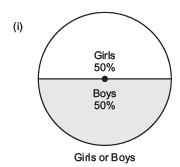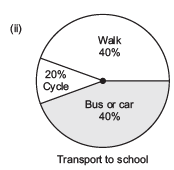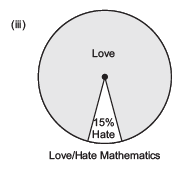Solution:

(i) Fraction of the circle representing the ‘girls’ 50% = 50/100 = 1/2
Fraction of the circle representing the ‘boys’ 50% = 50/100 = 1/2
(ii) Fraction of the circle representing ‘walk’ 40% = 40/100 =2/5
Fraction of the circle representing ‘bus or car’  40% = 40/100  = 2/5
Fraction of the circle representing ‘cycle’  20% =20/100 =1/5
(iii) Fraction of the circle representing those who love mathematics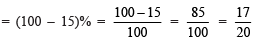Fraction of the circle representing those who hate mathematics = 15% = 15/100 = 3/20

Question 2. Answer the following questions based on the pie chart given.
(i) Which type of programmes are viewed the most?
(ii) Which two types of programmes have number of viewers equal to those watching sports channels?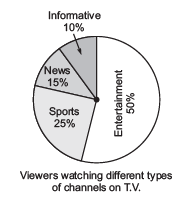Solution: From the given pie chart, we have

 Type of viewers Percentage Sports viewersNews viewersInformation viewersEntertainment viewers 25%15%10%50%

Obviously,

(i) The entertainment programmes are viewed the most.

(ii) The news and informative programmes have the equal number of viewers.

Question 3. Draw a pie chart of the data given below.

The time spent by a child during a day.

Sleep—8 hours

School—6 hours

Home work—4 hours

Play—4 hours

Others—2 hours

Solution: First we find the central angle corresponding to the given activities.

 Activity Duration of the activity in a day out of 24 hours Central angle corresponding to the activity Sleep 8 hours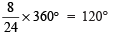School 6 hours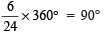Home work 4 hoursPlay 4 hour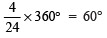Others 2 hours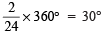Now, the required pie chart is given below: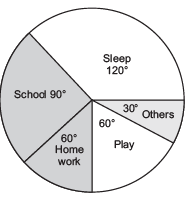Exercise 5.2

Question 1. A survey was made of find the type of music that a certain group of young people liked in a city. Adjoining pie chart shows the findings of this survey.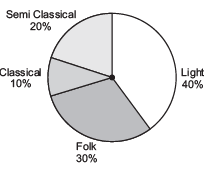From this pie chart answer the following:

(i) If 20 people liked classical music, how many young people were surveyed?

(ii) Which type of music is liked by the maximum number of people?

(iii) If a cassette company were to make 1000 CD’s how many of each type would they make?

Solution:

(i) Let the required number of young people = x

∴10% of x = 20

or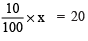or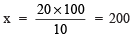(ii) Maximum number of people like the light music.

(iii) Total number of CD’s = 1000

∴Number of CD’s for semi classical = 20% of 1000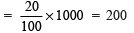Number of CD’s for classical = 10% of 1000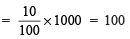Number of CD’s for folks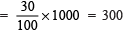Number of CD’s for light music = 40% of 1000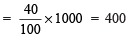Question 2. A group of 360 people were asked to vote for their favourite season from the three seasons rainy, winter and summer.

 Season No. of votes Summer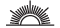Rainy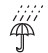Winter90120150

(i) Which season got the most votes?

(ii) Find the central angle of each sector.

(iii) Draw a pie chart to show this information.

Solution:

(i) Number of votes are maximum (150) for the winter season.

(ii) Total votes = 90 + 120 + 150 = 360

∴Central angle of the sector corresponding to:

Summer season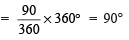Rainy season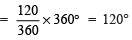Winter season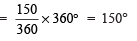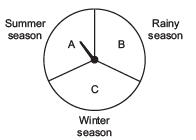Question 3. Draw a pie chart showing the following information. The table shows the colours preferred by a group of people.

 Colours Number of people BlueGreenRedYellow 18963 Total 36

Find the proportion of each sector. For example,
Blue is 18/36 = 1/2 ; Green is 9/36 =1/4 and so on.
Use this to find the corresponding angles.

Solution: Central angle of the sector corresponding to:

(a) The blue colour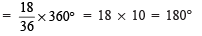(b) The green colour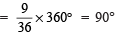(c) The red colour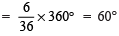(d) The yellow colour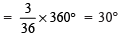Thus, the required pie chart is given below: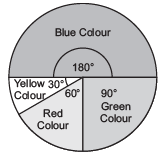Question 4. The adjoining pie chart gives the marks scored in an examination by a student in Hindi, English, Mathematics, Social Science and Science. If the total marks obtained by the student were 540, answer the following questions.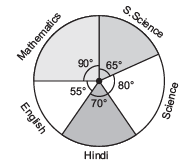(i) In which subject did the student score 105 marks?

Hint: for 540 marks, the central angle = 360°. So, for 105 marks, what is the central angle?

(ii) How many more marks were obtained by the student in Mathematics than in Hindi?

(iii) Examine whether the sum of the marks obtained in Social Science and Mathematics is more than that in Science and Hindi.

Hint: Just study the central angles.

Solution:

(i) Total marks = 540

∴ Central angle corresponding to 540 marks = 360°

Central angle corresponding to 105 marks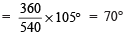Since the sector having central angle 70° is corresponding to Hindi.

Thus, the student obtained 105 marks in Hindi.

(ii) ∵ The central angle corresponding to the sector of Mathematics = 90°

∴Marks obtained in Mathematics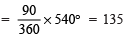Thus, marks more in Mathematics than in Hindi = 135 – 105 = 30

(iii) Since, the sum of the central angles for Social Science and Mathematics

= 65° + 90° = 155°

Also, the sum of the central angles for Science and Hindi

= 80° + 70° = 150°

∵ Marks obtained are proportional to the central angles corresponding to various items and 155° > 150°.

∵Marks of in Science and Mathematics are more than the marks obtained in Science and Hindi.

Question 5. The number of students in a hostel, speaking different languages is given below. Display the data in a pie chart.

 Language Hindi English Marathi Tamil Bengali Total Number of students 40 12 9 7 4 72

Solution: Central angle of the sector representing:

(a) Hindi language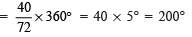(b) English language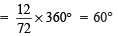(c) Marathi language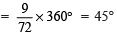(d) Tamil language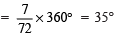(e) Bengali language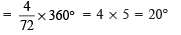Thus, the required pie chart is given below: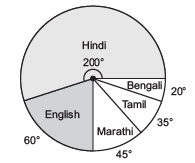Chance and Probability

Probability is the measure of the change of the happening of an event. The probability of an out come is represented by a number between 0 and 1.

Note: For an impossible event probability is 0 and for a certain event, the probability is 1.

Equally Likely Outcomes

When each of the outcomes of an experiment has the same chance of occurring, we say that it is equally likely outcome.

Example: A die is thrown. Number of the six outcomes are almost equal to each other, i.e. they are equally likely to occur.

Outcomes as Events

Each of the outcomes of an experiment or a collection of outcomes make an event.

For example:

(i) Throwing a die and getting each of the outcomes 1, 2, 3, 4, 5 or 6 is an event.
(ii) Tossing a coin and ‘getting a head’ or ‘getting a tail’ is an event.

Finding Probability

Question: (i) Let us toss a coin once. Then there are only two equally likely outcomes—Head or tail.

Since, Likely hood of getting a Head as an outcome out of the two outcomes.

∴ Probability of getting a Head = 1/2 =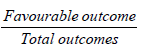Similarly,

Probability of getting a Tail = 1/2

(ii) A die is marked 1, 2 3, 4, 5 and 6 on its faces, such that one number is on one face. If we throw it once, the outcomes are 1, 2, 3, 4, 5 or 6.

∴ There are 6 equally likely outcomes.

So, probability of getting 4 as an outcome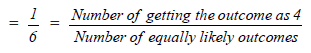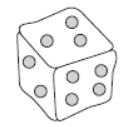Similarly, we get the probability of other numbers.

,

,

,

,

,

,

,

,

,

,

,

,

,

,

,

,

,

,

,

,

,

;